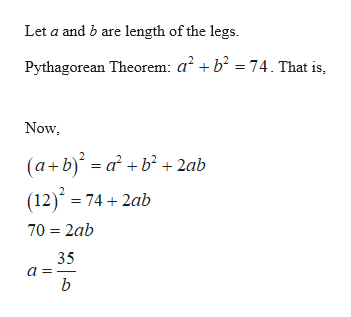# The hypotenuse of a right triangle is sqrt(74) ft and the sum of the lengths of the legs is 12 ft. Find the lengths of the legs.

Question
10 views

The hypotenuse of a right triangle is sqrt(74) ft and the sum of the lengths of the legs is 12 ft. Find the lengths of the legs.

check_circle

Step 1...help_outlineImage TranscriptioncloseLet a and b are length of the legs. Pythagorean Theorem: a? + b? = 74. That is, Now, (a+b) = a + b² + 2ab (12) = 74 + 2ab 70 = 2ab 35 a fullscreen

### Want to see the full answer?

See Solution

#### Want to see this answer and more?

Solutions are written by subject experts who are available 24/7. Questions are typically answered within 1 hour.*

See Solution
*Response times may vary by subject and question.
Tagged in

### Algebra